### 12.4 Examples

#### 12.4.1 L-shaped domain

The first example is the classic L-shaped domain, in which an exact solution is known, which makes it an ideal test case . The domain is the polygon formed from the vertices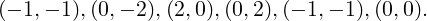The exact solution for the displacements is known in polar co-ordinates (r,θ) as

 ur(r,θ) =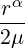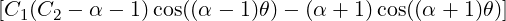uθ(r,θ) =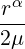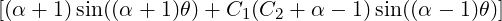where α ≈ 0.544483737… is the solution of the equation α sin(2ω) + sin(2ωα) = 0,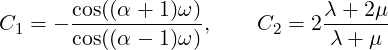with λ and μ being the Lamé constants and ω = 3π∕4. Boundary conditions are set to be the exact solution and f = 0. The solution has a singularity at the origin, and so in order to test convergence h-refinement is required.Figure 12.1: Solution of the u displacement field for the L-shaped domain.

A simple example of how the linear elastic solver can be set up can be found in the `Tests/L-shaped.xml` session file in the linear elastic solver directory. A more refined domain with the obtained u solution is shown in figure 12.1. The solver can be run using the command:

LinearElasticSolver L-domain.xml

The obtained solution `L-domain.fld` can be applied to the mesh to obtain a deformed XML file using the `deform` module in `FieldConvert`:

FieldConvert -m deform L-domain.xml L-domain.fld L-domain-deformed.xml

#### 12.4.2 Boundary layer deformation

In this example we use the iterative elastic system to apply a large deformation to a triangular boundary layer mesh of a square mesh Ω = [0,1]2. At the bottom edge, we apply a Dirichlet condition g =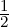sin(πx) that is enforced by splitting it into N substeps, so that at each step we solve the system with the boundary condition gn(x) = g(x)∕N. The process is depicted in figure 12.2.Figure 12.2: Figures that show the initial domain (left), after 50 steps (middle) and final deformation of the domain (right).

The setup is very straightforward. The geometry can be found inside the file `Examples/bl-mesh.xml` and the conditions inside `Examples/bl-conditions.xml`. The solver can be set up using the following parameters, with `NumSteps` denoting N:

1  <SOLVERINFO>
2    <I PROPERTY="EQTYPE" VALUE="IterativeElasticSystem" />
3  </SOLVERINFO>
4
5  <PARAMETERS>
6    <P> nu       = 0.3 </P>
7    <P> E        = 1.0 </P>
8    <P> NumSteps = 100 </P>
9  </PARAMETERS>

To identify the boundary that we intend to split up into substeps, we must assign the `WALL` tag to our boundary regions:

1  <BOUNDARYCONDITIONS>
2    <REGION REF="0">
3      <D VAR="u" VALUE="0" USERDEFINEDTYPE="Wall" />
4      <D VAR="v" VALUE="0.5*sin(PI*x)" USERDEFINEDTYPE="Wall" />
5    </REGION>
6    <REGION REF="1">
7      <D VAR="u" VALUE="0" />
8      <D VAR="v" VALUE="0" />
9    </REGION>
10  </BOUNDARYCONDITIONS>

The solver can then be run using the command:

LinearElasticSolver bl-mesh.xml bl-conditions.xml

This will produce a series of meshes `bl-mesh-%d.xml`, where %d is an integer running between 0 and 100. If at any point the mesh becomes invalid, that is, a negative Jacobian is detected, execution will cease.